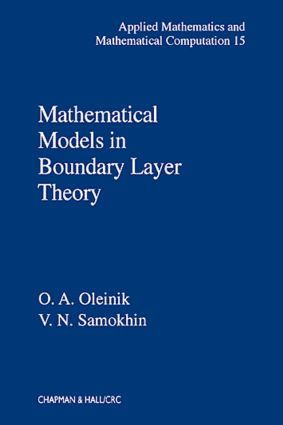# Mathematical Models in Boundary Layer Theory

## 1st Edition

Chapman and Hall/CRC

528 pages

##### Purchasing Options:\$ = USD
Hardback: 9781584880158
pub: 1999-05-25
SAVE ~\$37.00
\$185.00
\$148.00
x
eBook (VitalSource) : 9780203749364
pub: 2018-05-02
from \$28.98

FREE Standard Shipping!

### Description

Since Prandtl first suggested it in 1904, boundary layer theory has become a fundamental aspect of fluid dynamics. Although a vast literature exists for theoretical and experimental aspects of the theory, for the most part, mathematical studies can be found only in separate, scattered articles. Mathematical Models in Boundary Layer Theory offers the first systematic exposition of the mathematical methods and main results of the theory.

Beginning with the basics, the authors detail the techniques and results that reveal the nature of the equations that govern the flow within boundary layers and ultimately describe the laws underlying the motion of fluids with small viscosity. They investigate the questions of existence and uniqueness of solutions, the stability of solutions with respect to perturbations, and the qualitative behavior of solutions and their asymptotics. Of particular importance for applications, they present methods for an approximate solution of the Prandtl system and a subsequent evaluation of the rate of convergence of the approximations to the exact solution.

Written by the world's foremost experts on the subject, Mathematical Models in Boundary Layer Theory provides the opportunity to explore its mathematical studies and their importance to the nonlinear theory of viscous and electrically conducting flows, the theory of heat and mass transfer, and the dynamics of reactive and muliphase media. With the theory's importance to a wide variety of applications, applied mathematicians-especially those in fluid dynamics-along with engineers of aeronautical and ship design will undoubtedly welcome this authoritative, state-of-the-art treatise.

### Table of Contents

The Navier-Stokes Equations and Prandtl

Derivation of the Prandtl System

Solution of the Boundary Layer System as the First Approximation to Asymptotic Solution of the Navier-Stokes Equations near the Boundary

Separation of the Boundary Layer

Setting of the Main Problems for the Equations of Boundary Layer

Boundary Layer Equations for Non-Newtonian Fluids

Boundary Layers in Magnetohydrodynamics

Stationary Boundary Layer: von Mises Variables

Continuation of Two-Dimensional Boundary Layer

Asymptotic Behavior of the Velocity Component along the Boundary Layer

Conditions for Boundary Layer Separation

Self-Similar Solutions of the Boundary Layer Equations

Solving the Continuation Problem by the Line Method

On Three-Dimensional Boundary Layer Equations

Comments

Stationary Boundary Layer: Crocco Variables

Axially Symmetric Stationary Boundary Layer

Symmetric Boundary Layer

The Problem of Continuation of the Boundary Layer

Weak Solutions of the Boundary Layer System

Nonstationary Boundary Layer

Axially Symmetric Boundary Layer

The Continuation Problem for a Nonstationary Axially Symmetric Boundary Layer

Continuation of the Boundary Layer: Successive Approximations

On t-Global Solutions of the Prandtl System for Axially Symmetric Flows

Stability of Solutions of the Prandtl System

Time-Periodic Solutions of the Nonstationary Boundary Layer System

Solving the Nonstationary Prandtl System by the Line Method in the Time Variable

Formation of the Boundary Layer

Solutions and Asymptotic Expansions for the Problem of Boundary Layer formation: The Case of Gradual Acceleration

Formation of the Boundary Layer about a Body that Suddenly Starts to Move

Comments

Finite-Difference Method

Solving the Boundary Layer Continuation Problem by the Finite Difference Method

Solving the Prandtl System for Axially Symmetric Flows by the Finite Difference Method

Comments

Diffraction Problems for the Prandtl System

Boundary Layer with Unknown Border between Two media

Mixing of Two Fluids with Distinct Properties at the Interface between Two Flows

Comments

Boundary Layer in Non-Newtonian Flows

Symmetric Boundary Layer in Pseudo-Plastic Fluids

Weak Solutions of the Boundary Layer Continuation Problem for Pseudo-Plastic Fluids

Nonstationary Boundary Layer for Pseudo-Plastic Fluids

Continuation of the Boundary Layer in Dilatable Media

Symmetric Boundary Layer in Dilatable Media

Comments

Boundary Layer in Magnetic Hydrodynamics

Continuation of the MHD Boundary Layer in Ordinary Fluids

Solving the Equations of the MHD Boundary Layer in Pseudo-Plastic Fluids

Self-Similar Solutions of the MHD Boundary Layer System for a Dilatable Fluid

Solving the Equations of Boundary Layer for Dilatable Conducting Fluids in a Transversal Magnetic Field

Comments

Homogenization of Boundary Layer Equations

Homogenization of the Prandtl System with Rapidly Oscillating Injection and Suction

Homogenization of the Equations of the MHD Boundary Layer in a Rapidly Oscillating Magnetic Field

Comments

Some Open Problems

References

Index

### Subject Categories

##### BISAC Subject Codes/Headings:
MAT000000
MATHEMATICS / General
MAT007000
MATHEMATICS / Differential Equations
SCI040000
SCIENCE / Mathematical Physics